# Maharashtra Board Class 11 Chemistry Important Questions Chapter 6 Redox Reactions

Balbharti Maharashtra State Board 11th Chemistry Important Questions Chapter 6 Redox Reactions Important Questions and Answers.

## Maharashtra State Board 11th Chemistry Important Questions Chapter 6 Redox Reactions

Question 1.
What does the term ‘redox’ refer to?
Redox is an abbreviation used for the terms ‘oxidation and reduction’.

Question 2.
Give examples of naturally occurring redox reactions.

1. Respiration
2. Rusting
3. Combustion of fuel

Question 3.
Define Oxidant/Oxidising agent.
A reagent/substance which itself undergoes reduction and causes oxidation of another species is called oxidant/oxidising agent.

Question 4.
Define: Reductant/Reducing agent
A reagent/substance which itself undergoes oxidation bringing about the reduction of another species is called reductant/reducing agent.Question 5.
Explain redox reaction giving an example.
Oxidation and reduction reactions occur simultaneously. Therefore, the oxidation-reduction reaction is also referred to as a redox reaction.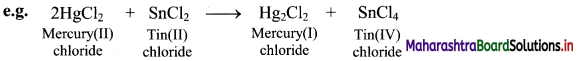In the above reaction, HgCl2 is reduced to Hg2Cl2 and SnCl2 is oxidised to SnCl4. Hence, it is a redox reaction.

Question 6.
Explain redox reaction in terms of electron transfer.
i. Redox reaction can be described in terms of electron transfer as shown below:
2Mg(s) + O2(g) → Mg2+ + 2O2-
ii. Charge development suggests that each magnesium atom loses two electrons to form Mg2+ and each oxygen atom gains two electrons to form O2-. This can be represented as follows: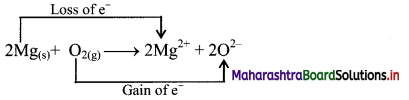iii. When Mg is oxidised to MgO, the neutral Mg atom loses electrons to form Mg2+ in MgO while the elemental oxygen gains electrons and forms O2- in MgO.
iv. Each of the above steps represents a half reaction which involves electron transfer (loss or gain).
v. Sum of these two half reactions or the overall reaction is a redox reaction.

Question 7.
Justify the following reaction as redox reaction in terms of electron transfer.
Mg + F2 → MgF2
i. Redox reaction can be described in terms of electron transfer as shown below:
Mg(s) + F2(g) → Mg2+ + 2F
ii. Charge development suggests that magnesium atom loses two electrons to form Mg2+ and each fluorine atom gains one electron to form F. This can be represented as follows: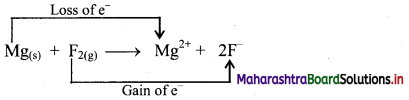iii. When Mg is oxidised to MgF2, the neutral Mg atom loses electrons to form Mg2+ in MgF2 while the elemental fluorine gains electrons and forms Fin MgF2.
iv. Each of the above steps represents a half reaction which involves electron transfer (loss or gain).
v. Sum of these two half reactions or the overall reaction is a redox reaction.

Question 8.
Justify that the reaction 2Na(s) + H2(g) → 2NaH(s) is a redox reaction.
Redox reaction can be described as electron transfer as shown below:
2Na(s) + H2(g) → 2Na+ + 2H
ii. Charge development suggests that each sodium atom loses one electron to form Na+ and each hydrogen atom gains one electron to form H. This can be represented as follows: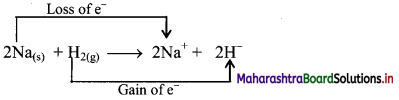iii. When Na is oxidised to NaH, the neutral Na atom loses one electron to form Na+ in NaH while the elemental hydrogen gains one electron and forms H in NaH.
iv. Each of the above steps represents a half reaction which involves electron transfer (loss or gain).
v. Sum of these two half reactions or the overall reaction is a redox reaction.

Question 9.
Define the terms oxidation and reduction in terms of electron transfer.
i. The half reaction involving loss of electrons is called oxidation reaction.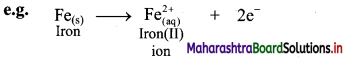ii. The half reaction involving gain of electrons is called reduction reaction.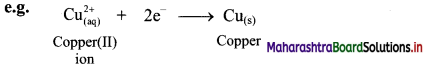Question 10.
Define the terms oxidant and reductant in terms of electron transfer.

1. Oxidant: Oxidant or oxidising agent is an electron acceptor.
2. Reductant: Reductant or reducing agent is an electron donor.Question 11.
Identify oxidising and reducing agents in the following reaction.
$$\mathrm{Fe}_{(s)}+\mathrm{Cu}_{(\mathrm{aq})}^{2+} \longrightarrow \mathrm{Fe}_{(\mathrm{aq})}^{2+}+\mathrm{Cu}_{(\mathrm{s})}$$
Fe(s) acts as a reducing agent as it donates electrons while $$\mathrm{Cu}_{(\mathrm{aq})}^{2+}$$ acts as an oxidising agent as it accepts electrons.

Question 12.
Define: Displacement reaction.
A reaction in which an ion (or an atom) in a compound is replaced by an ion (or an atom) of another element is called displacement reaction.
e.g. X + YZ → XZ + Y

Question 13.
Draw structure and assign oxidation number to each atom in:
i. Br3O8
ii. C3O2
i. Br3O8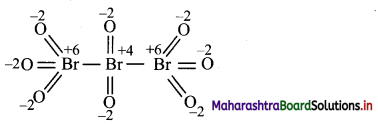ii. C3O2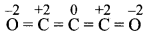Question 14.
Deduce the oxidation number of S in the following species:
i. SO2
ii. $$\mathrm{SO}_{4}^{2-}$$
i. SO2 is a neutral molecule.
∴ Sum of oxidation numbers of all atoms of SO2 = 0
∴ (Oxidation number of S) + 2 × (Oxidation number of O) = 0
∴ Oxidation number of S + 2 × (- 2) = 0
∴ Oxidation number of S in SO2 = 0 – (- 4)
∴ Oxidation number of S in SO2 = +4

ii. $$\mathrm{SO}_{4}^{2-}$$ is an ionic species.
∴ Sum of the oxidation numbers of all atoms of $$\mathrm{SO}_{4}^{2-}$$ = – 2
∴ (Oxidation number of S) + 4 × (Oxidation number of O) = – 2
∴ Oxidation number of S in $$\mathrm{SO}_{4}^{2-}$$ = – 2 – 4 × (-2) = – 2 + 8
∴ Oxidation number of S in $$\mathrm{SO}_{4}^{2-}$$ = +6Question 15.
Assign oxidation number to each element in the following compounds or ions.
i. KMnO4
ii. K2Cr2O7
iii. Ca3(PO4)2
i. KMnO4
Oxidation number of K = +1
Oxidation number of O = -2
KMnO4 is a neutral molecule.
∴ Sum of the oxidation numbers of all atoms = 0
∴ (Oxidation number of K) + (Oxidation number of Mn) + 4 × (Oxidation number of O) = 0
∴ (+1) + Oxidation number of Mn + 4 × (-2) = 0
∴ Oxidation number of Mn + 1 – 8 = 0
∴ Oxidation number of Mn – 7 = 0
∴ Oxidation number of Mn in KMnO4 = +7

ii. K2Cr2O7
Oxidation number of K = +1
Oxidation number of O = -2
K2Cr2O7 is a neutral molecule.
∴ Sum of the oxidation numbers of all atoms = 0
∴ 2 × (Oxidation number of K) + 2 × (Oxidation number of Cr) + 7 × (Oxidation number of O) = 0
∴ 2 × (+1) + 2 × (Oxidation number of Cr) + 7 × (-2) = 0
∴ 2 × (Oxidation number of Cr) + 2 – 14 = 0
∴ 2 × (Oxidation number of Cr) – 12 = 0
∴ 2 × (Oxidation number of Cr) = +12
∴ Oxidation number of Cr = +12/2
∴ Oxidation number of Cr in K2Cr2O7 = +6

iii. Ca3(PO4)2
Oxidation number of Ca = +2 (∵ Ca is alkaline earth metal.)
Oxidation number of O = -2
Ca3(PO4)2 is a neutral molecule.
Sum of the oxidation numbers of all atoms = 0
∴ 3 × (Oxidation number of Ca) + 2 × (Oxidation number of P) + 8 × (Oxidation number of O) = 0
∴ 3 × (+2)+ 2 × (Oxidation number of P)+ 8 × (-2) = 0
∴ 2 × (Oxidation number of P) + 6 – 16 = 0
∴ 2 × (Oxidation number of P) – 10 = 0
∴ 2 × (Oxidation number of P) = +10
∴ Oxidation number of P = +10/2
∴ Oxidation number of P in Ca3(PO4)2 = +5

Question 16.
Assign oxidation number to the atoms other than O and H in the following species.
i. $$\mathrm{SO}_{3}^{2-}$$
ii. $$\mathrm{BrO}_{3}^{-}$$
iii. $$\mathrm{ClO}_{4}^{-}$$
iv. $$\mathrm{NH}_{4}^{+}$$
v. $$\mathrm{NO}_{3}^{-}$$
vi. $$\mathrm{NO}_{2}^{-}$$
vii. SO3
viii. N2O5
The oxidation number of O atom bonded to a more electropositive atom is -2 and that of H atom bonded to electronegative atom is +1. Sum of the oxidation numbers of all atoms in ionic species is equal to charge it carries and that for neutral molecule is zero. Using these values, the oxidation numbers of atoms of the other elements in a given polyatomic species are calculated as follows:
i. $$\mathrm{SO}_{3}^{2-}$$
(Oxidation number of S) + 3 × (Oxidation number of O) = – 2
∴ Oxidation number of S + 3 × (-2) = – 2
∴ Oxidation number of S – 6 = – 2
∴ Oxidation number of S = – 2 + 6
∴ Oxidation number of S in $$\mathrm{SO}_{3}^{2-}$$ = +4

ii. $$\mathrm{BrO}_{3}^{-}$$
(Oxidation number of Br) + 3 × (Oxidation number of O) = -1
∴ Oxidation number of Br + 3 × (-2) = – 1
∴ Oxidation number of Br – 6 = – 1
∴ Oxidation number of Br = – 1 + 6
Oxidation number of Br in $$\mathrm{BrO}_{3}^{-}$$ = +5

iii. $$\mathrm{ClO}_{4}^{-}$$
(Oxidation number of Cl) + 4 × (Oxidation number of O) = – 1
∴ Oxidation number of Cl + 4 × (-2) = – 1
∴ Oxidation number of Cl – 8 = – 1
∴ Oxidation number of Cl = – 1 + 8
∴ Oxidation number of Cl in $$\mathrm{ClO}_{4}^{-}$$ = +7

iv. $$\mathrm{NH}_{4}^{+}$$
(Oxidation number of N) + 4 × (Oxidation number of H) = + 1
∴ Oxidation number of N + 4 × (+1) = +1
∴ Oxidation number of N + 4 = + 1
∴ Oxidation number of N = + 1 – 4
∴ Oxidation number of N in $$\mathrm{NH}_{4}^{+}$$ = -3

v. $$\mathrm{NO}_{3}^{-}$$
(Oxidation number of N) + 3 × (Oxidation number of O) = – 1
∴ Oxidation number of N + 3 × (-2) = – 1
∴ Oxidation number of N – 6 = – 1
∴ Oxidation number of N = – 1 + 6
∴ Oxidation number of N in $$\mathrm{NO}_{3}^{-}$$ = +5

vi. $$\mathrm{NO}_{2}^{-}$$
(Oxidation number of N) + 2 × (Oxidation number of O) = – 1
∴ Oxidation number of N + 2 × (-2) = – 1
∴ Oxidation number of N – 4 = – 1
∴ Oxidation number of N = – 1 + 4
∴ Oxidation number of N in $$\mathrm{NO}_{2}^{-}$$ = +3

vii. SO3
(Oxidation number of S) + 3 × (Oxidation number of O) = 0
∴ Oxidation number of S + 3 × (-2) = 0
∴ Oxidation number of S – 6 = 0
∴ Oxidation number of S = 0 + 6
∴ Oxidation number of S in SO3 = +6

viii. N2O5
2 × (Oxidation number of N) + 5 × (Oxidation number of O) = 0
∴ 2 × (Oxidation number of N) + 5 × (-2) = 0
∴ 2 × (Oxidation number of N) – 10 = 0
∴ 2 × (Oxidation number of N) = 0 + 10
∴ Oxidation number of N = 10/2
∴ Oxidation number of N in N2O5 = +5

Question 17.
Find the oxidation numbers of the underlined species in the following compounds or ions.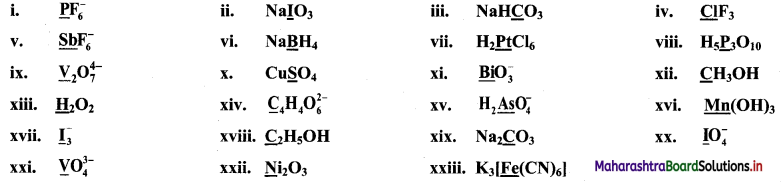i. P$$\mathrm{F}_{6}^{-}$$
Oxidation number of F = -1
P$$\mathrm{F}_{6}^{-}$$ is an ionic species.
∴ Sum of the oxidation numbers of all atoms = – 1
∴ (Oxidation number of P) + 6 × (Oxidation number of F) = – 1
∴ Oxidation number of P + 6 × (-1) = -1
∴ Oxidation number of P – 6 = – 1
Oxidation number of P in P$$\mathrm{F}_{6}^{-}$$ = +5

ii. NaIO3
Oxidation number of Na = +1
Oxidation number of O = -2
NaIO3 is a neutral molecule.
∴ Sum of the oxidation numbers of all atoms = 0
(Oxidation number of Na) + (Oxidation number of I) + 3 × (Oxidation number of O) = 0
(+1) + (Oxidation number of I) + 3 × (-2) = 0
Oxidation number of I + 1 – 6 = 0
Oxidation number of I in NaIO3 = +5

iii. NaHCO3
Oxidation number of Na = +1
Oxidation number of H = +1
Oxidation number of O = -2
NaHCO3 is a neutral molecule.
∴ Sum of the oxidation numbers of all atoms = 0
∴ (Oxidation number of Na) + (Oxidation number of H) + (Oxidation number of C) + 3 × (Oxidation number of O) = 0
∴ (+1) + (+1) + (Oxidation number of C) + 3 × (-2) = 0
∴ Oxidation number of C + 2 – 6 = 0
∴ Oxidation number of C in NaHCO3 = +4

iv. ClF3
Oxidation number of F = -1
ClF3 is a neutral molecule.
∴ Sum of the oxidation numbers of all atoms = 0
∴ (Oxidation number of Cl) + 3 × (Oxidation number of F) = 0
∴ Oxidation number of Cl + 3 × (-1) = 0
∴ Oxidation number of Cl in ClF3 = +3

v. Sb$$\mathrm{F}_{6}^{-}$$
Oxidation number of F = -1
Sb$$\mathrm{F}_{6}^{-}$$ is an ionic species.
∴ Sum of the oxidation numbers of all atoms = – 1
∴ (Oxidation number of Sb) + 6 × (Oxidation number of F) = – 1
∴ Oxidation number of Sb + 6 × (-1) = -1
∴ Oxidation number of Sb in Sb$$\mathrm{F}_{6}^{-}$$ = +5

vi. NaBH4
Oxidation number of Na =+1
Oxidation number of H = -1 (for Hydride)
NaBH4 is a neutral molecule.
∴ Sum of the oxidation numbers of all atoms = 0
∴ (Oxidation number of Na) + (Oxidation number of B) + 4 × (Oxidation number of H) = 0
∴ (+1) + (Oxidation number of B) + 4 × (-1) = 0
∴ Oxidation number of B + 1 – 4 = 0
∴ Oxidation number of B in NaBH4 = +3

vii. H2PtCl6
Oxidation number of H = +1
Oxidation number of Cl = -1
H2PtCl6 is a neutral molecule.
∴ Sum of the oxidation numbers of all atoms = 0
∴ 2 × (Oxidation number of H) + (Oxidation number of Pt) + 6 × (Oxidation number of Cl) = 0
∴ 2 × (+1) + (Oxidation number of Pt) + 6 × (-1) = 0
(Oxidation number of Pt) + 2 – 6 = 0
∴ Oxidation number of Pt in H2PtCl6 = +4

viii. H5P3O10
Oxidation number of H = +1
Oxidation number of O = -2
H5P3O10 is a neutral molecule.
∴ Sum of the oxidation numbers of all atoms = 0
∴ 5 × (Oxidation number of H) + 3 × (Oxidation number of P) +10 × (Oxidation number of O) = 0
∴ 5 × (+1) + 3 × (Oxidation number of P) + 10 × (-2) = 0
∴ 3 × (Oxidation number of P) + 5 – 20 = 0
Oxidation number of P = +$$\frac {15}{3}$$
∴ Oxidation number of P in H5P3O10 = +5

ix. V2$$\mathrm{O}_{7}^{4-}$$
Oxidation number of O = -2
V2$$\mathrm{O}_{7}^{4-}$$ is an ionic species.
∴ Sum of the oxidation numbers of all atoms = – 4
∴ 2 × (Oxidation number of V) + 7 × (Oxidation number of O) = – 4
∴ 2 × (Oxidation number of V) + 7 × (-2) = – 4
∴ 2 × (Oxidation number of V) = – 4 + 14
∴ Oxidation number of V = +$$\frac {10}{2}$$
∴ Oxidation number of V in V2$$\mathrm{O}_{7}^{4-}$$ = +5

x. CuSO4
Oxidation number of Cu = +2
Oxidation number of O = -2
CuSO4 is a neutral molecule.
∴ Sum of the oxidation numbers of all atoms = 0
∴ (Oxidation number of Cu) + (Oxidation number of S) + 4 × (Oxidation number of O) = 0
∴ (+2) + Oxidation number of S + 4 × (-2) = 0
∴ Oxidation number of S + 2 – 8 = 0
∴ Oxidation number of S in CuSO4 = +6

xi. Bi$$\mathrm{O}_{3}^{-}$$
Oxidation number of O = -2
Bi$$\mathrm{O}_{3}^{-}$$ is an ionic species.
∴ Sum of the oxidation numbers of all atoms = – 1
∴ (Oxidation number of Bi) + 3 × (Oxidation number of O) = – 1
∴ Oxidation number of Bi + 3 × (-2) = – 1
∴ Oxidation number of Bi = – 1 + 6
∴ Oxidation number of Bi in Bi$$\mathrm{O}_{3}^{-}$$ = +5

xii. CH3OH
Oxidation number of H = +1
Oxidation number of O = -2
CH3OH is a neutral molecule.
∴ Sum of the oxidation numbers of all atoms = 0
∴ (Oxidation number of C) + 4 × (Oxidation number of H) + (Oxidation number of O) = 0
∴ (Oxidation number of C) + 4 × (+1) + (-2) = 0
∴ Oxidation number of C + 2 = 0
∴ Oxidation number of C in CH3OH = -2

xiii. H2O2
Oxidation number of O = -1 (for peroxide)
H2O2 is a neutral molecule.
∴ Sum of the oxidation numbers of all atoms = 0
∴ 2 × (Oxidation number of H) + 2 × (Oxidation number of O) = 0
∴ 2 × (Oxidation number of H) + 2 × (-1) = 0
∴ Oxidation number of H = +$$\frac {2}{2}$$
∴ Oxidation number of H in H2O2 = +1

xiv. C4H4$$\mathrm{O}_{6}^{2-}$$
Oxidation number of H = +1
Oxidation number of O = -2
C4H4$$\mathrm{O}_{6}^{2-}$$ is an ionic species.
∴ Sum of the oxidation numbers of all atoms = – 2
∴ 4 × (Oxidation number of C) + 4 × (Oxidation number of H) + 6 × (Oxidation number of O) = – 2
∴ 4 × (Oxidationnumber of C) + 4 × (+1) +6 × (-2) = -2
∴ 4 × (Oxidation number of C) + 4 – 12 = -2
∴ 4 × (Oxidation number of C) = – 2 + 8
∴ Oxidation number of C = +$$\frac {6}{4}$$
∴ Oxidation number of C in C4H4$$\mathrm{O}_{6}^{2-}$$ = +1.5

xv. H2As$$\mathrm{O}_{4}^{-}$$
Oxidation number of H = +1
Oxidation number of O = -2
H2As$$\mathrm{O}_{4}^{-}$$ is an ionic species.
∴ Sum of the oxidation numbers of all atoms = – 1
∴ 2 × (Oxidation number of H) + (Oxidation number of As) + 4 × (Oxidation number of O) = -1
∴ 2 × (+1) + Oxidation number of As + 4 × (-2) = – 1
∴ Oxidation number of As + 2 – 8 = – 1
∴ Oxidation number of As = – 1 + 6
∴ Oxidation number of As in H2As$$\mathrm{O}_{4}^{-}$$ = +5

xvi. Mn(OH)3
Oxidation number of O = -2
Oxidation number of H = +1
Mn(OH)3 is a neutral molecule.
∴ Sum of the oxidation numbers of all atoms = 0
∴ (Oxidation number of Mn) + 3 × (Oxidation number of O) + 3 × (Oxidation number of H) = 0
∴ Oxidation number of Mn + 3 × (-2) + 3 × (+1) = 0
∴ Oxidation number of Mn – 6 + 3 = 0
∴ Oxidation number of Mn in Mn(OH)3 = +3

xvii. $$\mathrm{I}_{3}^{-}$$
$$\mathrm{I}_{3}^{-}$$ is an ionic species.
∴ Sum of the oxidation numbers of all atoms = – 1
∴ 3 × Oxidation number of I = – 1
∴ Oxidation number of I in $$\mathrm{I}_{3}^{-}$$ = –$$\frac {1}{3}$$

xviii. C2H5OH
Oxidation number of O = -2
Oxidation number of H = +1
C2H5OH is a neutral molecule.
∴ Sum of the oxidation numbers of all atoms = 0
∴ 2 × (Oxidation number of C) + 6 × (Oxidation number of H) + (Oxidation number of O) = 0
∴ 2 × (Oxidationnumberof C) + 6 × (+1) + (-2) = 0
∴ 2 × (Oxidation number of C) = – 4
∴ Oxidation number of C = –$$\frac {4}{2}$$
∴ Oxidation number of C in C2H5OH = -2

xix. Na2CO3
Oxidation number of Na = +1
Oxidation number of O = -2
Na2CO3 is a neutral molecule.
∴ Sum of the oxidation numbers of all atoms = 0
∴ 2 × (Oxidation number of Na) + (Oxidation number of C) + 3 × (Oxidation number of O) = 0
∴ 2 × (+1) + (Oxidation number of C) + 3 × (-2) = 0
∴ Oxidation number of C + 2 – 6 = 0
∴ Oxidation number of C in Na2CO3 = +4

xx. I$\mathrm{O}_{4}^{-}$
Oxidation number of O = -2
I$$\mathrm{O}_{4}^{-}$$ is an ionic species.
∴ Sum of the oxidation numbers of all atoms = – 1
∴ (Oxidation number of I) + 4 × (Oxidation number of O) = – 1
∴ Oxidation number of I + 4 × (-2) = – 1
∴ Oxidation number of I = -1 +8
∴ Oxidation number of I in I$$\mathrm{O}_{4}^{-}$$ = +7

xxi. V$$\mathrm{O}_{4}^{3-}$$
Oxidation number of O = -2
V$$\mathrm{O}_{4}^{3-}$$ is an ionic species.
∴ Sum of the oxidation numbers of all atoms = – 3
∴ (Oxidation number of V) + 4 × (Oxidation number of O) = – 3
∴ Oxidation number of V + 4 × (-2) = – 3
∴ Oxidation number of V = -3 + 8
∴ Oxidation number of V in V$$\mathrm{O}_{4}^{3-}$$ = +5

xxii. Ni2O3
Oxidation number of O = -2
Ni2O3 is a neutral molecule.
∴ Sum of the oxidation numbers of all atoms = 0
∴ 2 × (Oxidation number of Ni) + 3 × (Oxidation number of O) = 0
∴ 2 × (Oxidation number of Ni) + 3 × (-2) = 0
∴ 2 × (Oxidation number of Ni) = +6
∴ Oxidation number of Ni = +$$\frac {6}{2}$$
∴ Oxidation number of Ni in Ni2O3 = +3

xxiii. K3[Fe(CN)6]
Oxidation number of K = +1
Oxidation number of CN group = -1
K3[Fe(CN)6] is a neutral molecule.
∴ Sum of the oxidation numbers of all atoms = 0
∴ 3 × (Oxidation number of K) + (Oxidation number of Fe) + 6 × (Oxidation number of CN group) = 0
∴ 3 × (+1) + Oxidation number of Fe + 6 × (-1) = O
∴ Oxidation number of Fe + 3 – 6 = 0
∴ Oxidation number of Fe in K3[Fe(CN)6] = +3Question 18.
Define: Stock notation.
Representation in which oxidation number of an atom is denoted by Roman numeral in parentheses after the chemical symbol is called Stock notation. This name was given after the German scientist, Alfred Stock. e.g. Au1+Cl1- → Au(I)Cl

Question 19.
What is the use of Stock notation?
The Stock notation is used to specify the oxidation number of the metal.

Question 20.
How will you write Stock notations for the following compounds?
i. AuCl3
ii. SnCl4
iii. SnCl2
iv. MnO2
i. AuCl3: The charge on each element is $$\mathrm{Au}^{3+} \mathrm{Cl}_{3}^{1-}$$. Hence, the stock notation is Au(III)Cl3.
ii. SnCl4: The charge on each element is $$\mathrm{Sn}^{4+} \mathrm{Cl}_{4}^{1-}$$. Hence, the stock notation is Sn(IV)Cl4.
iii. SnCl2: The charge on each element is $$\mathrm{Sn}^{2+} \mathrm{Cl}_{2}^{1-}$$. Hence, the stock notation is Sn(II)Cl2.
iv. MnO2: The charge on each element is $$\mathrm{Mn}^{4+} \mathrm{O}_{2}^{2-}$$. Hence, the stock notation is Mn(IV)O2.

Question 21.
Write the formula for each of the following ionic compounds:
i. Nickel(III) oxide
ii. Tin(IV) chloride
iii. Bismuth(V) chloride
iv. Cobalt(III) chloride
vi. Chromium(II) chloride
i. Ni2O3
ii. SnCl4
iii. BiCl5
iv. CoCl3
v. PbO2
vi. CrCl2

Question 22.
Define the terms oxidation and reduction in terms of oxidation number.
i. Oxidation is an increase in the oxidation number of an element in a given substance.
e.g. Fe(s) → $$\mathrm{Fe}_{(\mathrm{aq})}^{2+}$$
ii. Reduction is a decrease in the oxidation number of an element in a given substance. e.g. $$\mathrm{Cu}_{(\mathrm{aq})}^{2+}$$ → Cu(s)Question 23.
Define the terms oxidant and reductant in terms of oxidation number.

1. Oxidant: Oxidant or oxidising agent is a substance which increases the oxidation number of an element in a given substance, and itself undergoes decrease in oxidation number of a constituent element in it.
2. Reductant: Reductant or reducing agent is a substance that lowers the oxidation number of an element in a given substance, and itself undergoes an increase in the oxidation number of a constituent element in it.

Question 24.
Identify whether the following reaction is redox or NOT. State oxidant and reductant therein.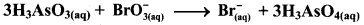i. Write oxidation number of all the atoms of reactants and products.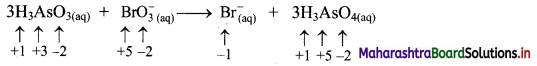ii. Identify the species that undergoes change in oxidation number.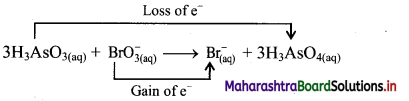iii. The oxidation number of As increases from +3 to +5 and that of Br decreases from +5 to -1. Because oxidation number of one species increases and that of the other decreases, the reaction is a redox reaction.
iv. The oxidation number of As increases by loss of electrons and therefore, As is a reducing agent and itself is oxidised. On the other hand, the oxidation number of Br decreases and therefore, Br is an oxidising agent and itself is reduced by gain of electrons.
Result:
a. The given reaction is a redox reaction.
b. Oxidant/oxidising agent: $$\mathrm{BrO}_{3}^{-}$$
c. Reductant/reducing agent: H3AsO3

Question 25.
For the reaction, $$\mathrm{SeO}_{3(\mathrm{aq})}^{2-}+\mathrm{Cl}_{2(\mathrm{~g})}+2 \mathrm{OH}_{(\mathrm{aq})}^{-} \longrightarrow \mathrm{SeO}_{4(\mathrm{aq})}^{2-}+2 \mathrm{Cl}_{(\mathrm{aq})}^{-}+\mathrm{H}_{2} \mathrm{O}_{(l)}$$, complete the following table.

 Oxidising agent ————– Reducing agent ————– Oxidised species ————– Reduced species ————–

 Oxidising agent Cl2(g) Reducing agent $$\mathrm{Se} \mathrm{O}_{3(\mathrm{aq})}^{2-}$$ Oxidised species $$\mathrm{Se} \mathrm{O}_{4(\mathrm{aq})}^{2-}$$ Reduced species $$2 \mathrm{Cl}_{(\mathrm{aq})}^{-}$$

Question 26.
Using oxidation number concept, identify the redox reactions, identify oxidizing and reducing agents in case of redox reactions.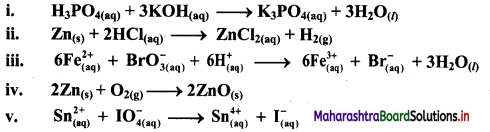i. H3PO4(aq) + 3KOH(aq) → K3PO4(aq) + 3H2O(l)
a. Write oxidation number of all the atoms of reactants and products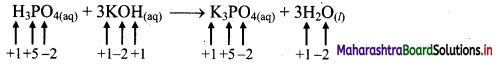b. Since, the oxidation numbers of all the species remain same, this is NOT a redox reaction.

Result:
The given reaction is NOT a redox reaction.

ii. Zn(s) + 2HCl(aq) → ZnCl2((aq)) + H2(g)
a. Write oxidation number of all the atoms of reactants and products.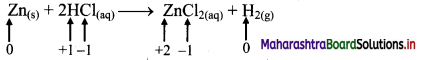b. Identify the species that undergoes change in oxidation number.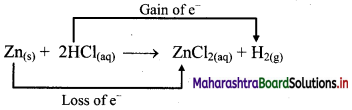c. The oxidation number of Zn increases from 0 to +2 and that of H decreases from +1 to 0. Because oxidation number of one species increases and that of other decreases, the reaction is redox reaction.
d. The oxidation number of Zn increases by loss of electrons and therefore, Zn is a reducing agent and itself is oxidized. On the other hand, the oxidation number of H decreases by gain of electrons and therefore, H is oxidising an agent and itself is reduced by gain of electrons.

Result:

1. The given reaction is a redox reaction.
2. Oxidant/oxidising agent: HCl
3. Reductant/reducing agent: Zn

iii.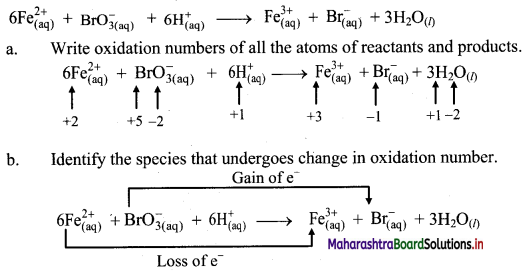c. The oxidation number of Fe increases from +2 to +3 and that of Br decreases from +5 to -1. Because oxidation number of one species increases and that of the other decreases, the reaction is a redox reaction.
d. The oxidation number of Fe increases by loss of electrons and therefore, Fe is a reducing agent and itself is oxidized. On the other hand, the oxidation number of Br decreases by gain of electrons and therefore, Br is an oxidising an agent and itself is reduced.

Result:

1. The given reaction is a redox reaction.
2. Oxidant/oxidising agent: $$\mathrm{BrO}_{3}^{-}$$
3. Reductant/reducing agent: Fe2+

iv. 2Zn(s) + O2(g) → 2ZnO(s)
a. Write oxidation number of all the atoms of reactants and products.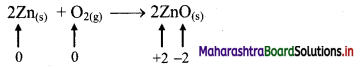b. Identify the species that undergoes change in oxidation number.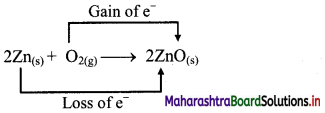c. The oxidation number of Zn increases from 0 to +2 and that of O decreases from 0 to -2. Because oxidation number of one species increases and that of the other decreases, the reaction is a redox reaction.
d. The oxidation number of Zn increases by loss of electrons and therefore, Zn is a reducing agent and itself is oxidized. On the other hand, the oxidation number of O decreases by gain of electrons and therefore, O is an oxidising agent and itself is reduced.

Result:

1. The given reaction is a redox reaction.
2. Oxidant/oxidising agent: O2
3. Reductant/reducing agent: Zn

v. $$\mathrm{Sn}_{(a q)}^{2+}+\mathrm{IO}_{4(2 q)}^{-} \longrightarrow \mathrm{Sn}_{(aq)}^{4+}+\mathrm{I}_{(a \mathrm{q})}^{-}$$
a. Write oxidation number of all the atoms of reactants and products.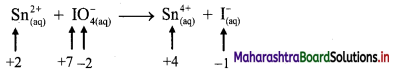b. Identify the species that undergoes change in oxidation number.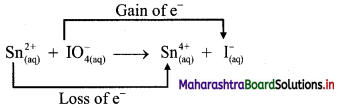c. The oxidation number of Sn increases from +2 to +4 and that of I decreases from +7 to -1. Because oxidation number of one species increases and that of the other decreases, the reaction is a redox reaction.
d. The oxidation number of Sn increases by loss of electrons and therefore, Sn is a reducing agent and itself is oxidized. On the other hand, the oxidation number of I decreases by gain of electrons and therefore, I is an oxidising agent and itself is reduced.

Result:

1. The given reaction is a redox reaction.
2. Oxidant/oxidising agent: Sn2+
3. Reductant/reducing agent: $$\mathrm{IO}_{4}^{-}$$Question 27.
Name two methods used to balance redox reactions.

1. Oxidation number method
2. Half reaction method or ion electrode method

Question 28.
Describe the steps involved in balancing redox reactions by the oxidation number method.
i. Step 1: Write the unbalanced equation for redox reaction. Balance the equation for all atoms in the reactions, except H and O. Identify the atoms which undergo change in oxidation number and by how much. Draw the bracket to connect atoms of the elements that changes the oxidation number.

ii. Step 2: Show an increase in oxidation number per atom of the oxidised species and hence, the net increase in oxidation number. Similarly, show a decrease in the oxidation number per atom of the reduced species and the net decrease in oxidation number. Determine the factors which will make the total increase and decrease equal. Insert the coefficients into the equation.

iii. Step 3: Balance oxygen atoms by adding H2O to the side containing less O atoms, one H2O is added for one O atom. Balance H atoms by adding H+ ions to the side having less H atoms.

iv. Step 4: If the reaction occurs in basic medium, then add OH ions equal to the number of H+ ions added in step 3, on both the sides of equation. The H+ and OH ions on same side of reactions are combined to give H2O molecules.

v. Step 5: Check the equation with respect to both, the number of atoms of each element and the charges. It is balanced.
Note: For acidic medium, step 4 is omitted.

Question 29.
Using the oxidation number method write the net ionic equation for the reaction of potassium permanganate, KMnO4, with ferrous sulphate, FeSO4.
$$\mathrm{MnO}_{4(\mathrm{aq})}^{-}+\mathrm{Fe}_{(\mathrm{aq})}^{2+} \longrightarrow \mathrm{Mn}_{(\mathrm{aq})}^{2+}+\mathrm{Fe}_{(\mathrm{aq})}^{3+}$$
Step 1: Write skeletal equation and balance the elements other than O and H.
$$\mathrm{MnO}_{4(\mathrm{aq})}^{-}+\mathrm{Fe}_{(\mathrm{aq})}^{2+} \longrightarrow \mathrm{Mn}_{(\mathrm{aq})}^{2+}+\mathrm{Fe}_{(\mathrm{aq})}^{3+}$$

Step 2: Assign oxidation number to Mn and Fe, and calculate the increase and decrease in the oxidation number and make them equal.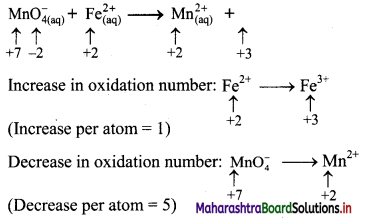To make the net increase and dicrease equal, we must take 5 atoms of Fe2+
$$\mathrm{MnO}_{4(\mathrm{aq})}^{-}+5 \mathrm{Fe}_{(\mathrm{aq})}^{2+} \longrightarrow \mathrm{Mn}_{(\mathrm{aq})}^{2+}+5 \mathrm{Fe}_{(\mathrm{aq})}^{3+}$$

Step 3: Balance the ‘O’ atoms by adding 4H2O to the right-hand side.
$$\mathrm{MnO}_{4(\mathrm{aq})}^{-}+5 \mathrm{Fe}_{(\mathrm{aq})}^{2+} \longrightarrow \mathrm{Mn}_{(\mathrm{aq})}^{2+}+5 \mathrm{Fe}_{(\mathrm{aq})}^{3+}+4 \mathrm{H}_{2} \mathrm{O}_{(l)}$$

Step 4: The medium is acidic. To make the charges and hydrogen atoms on the two sides equal, add 8H+ on the left-hand side.
$$\mathrm{MnO}_{4(\mathrm{aq})}^{-}+5 \mathrm{Fe}_{(\mathrm{aq})}^{2+}+8 \mathrm{H}_{(\mathrm{aq})}^{+} \longrightarrow \mathrm{Mn}_{(\mathrm{aq})}^{2+}+5 \mathrm{Fe}_{(\mathrm{aq})}^{3+}+4 \mathrm{H}_{2} \mathrm{O}_{(l)}$$

Step 5: Check the two sides for balance of charges and atoms. The net ionic equation obtained in step 4 is the balanced equation.
Hence, balanced equation:
$$\mathrm{MnO}_{4(\mathrm{aq})}^{-}+5 \mathrm{Fe}_{(\mathrm{aq})}^{2+}+8 \mathrm{H}_{(\mathrm{aq})}^{+} \longrightarrow \mathrm{Mn}_{(\mathrm{aq})}^{2+}+5 \mathrm{Fe}_{(\mathrm{aq})}^{3+}+4 \mathrm{H}_{2} \mathrm{O}_{(l)}$$Question 30.
Balance the following reaction by oxidation number method.
CuO + NH3 → Cu + N2 + H2O
Step 1: Write skeletal equation and balance the elements other than O and H.
CuO + 2NH3 → Cu + N2 + H2O
Step 2: Assign oxidation number to Cu and N. Calculate the increase and decrease in the oxidation number and make them equal.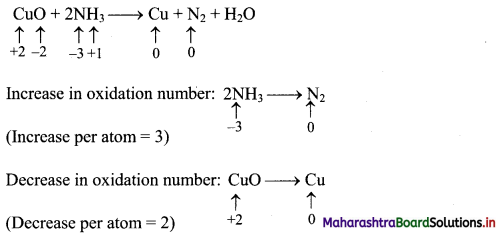To make the net increase and decrease equal, we must take 3 atoms of Cu and 2 atoms of N. (There are already 2 N atoms.)
3CuO + 2NH3 → 3Cu + N2 + H2O
Step 3: Balance ‘O’ atoms by adding 3H2O to the right-hand side.
3CuO + 2NH3 → 3Cu + N2 + 3H2O
Step 4: Charges are already balanced.
Step 5: Check two sides for balance of atoms and charges. The equation obtained in step 3 is balanced.
Hence, balanced equation: 3CuO + 2NH3 → 3Cu + N2 + 3H2O

Question 31.
Balance the following redox equation by oxidation number method. The reactions occur in acidic medium.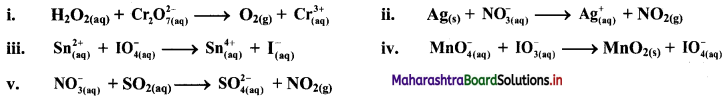i. $$\mathrm{H}_{2} \mathrm{O}_{2(\mathrm{aq})}+\mathrm{Cr}_{2} \mathrm{O}_{7 \text { (aq) }}^{2-} \longrightarrow \mathrm{O}_{2(\mathrm{~g})}+\mathrm{Cr}_{(\mathrm{aq})}^{3+}$$
Step 1: Write skeletal equation and balance the elements other than O and H.
$$\mathrm{H}_{2} \mathrm{O}_{2(\mathrm{aq})}+\mathrm{Cr}_{2} \mathrm{O}_{7 \text { (aq) }}^{2-} \longrightarrow \mathrm{O}_{2(\mathrm{~g})}+2 \mathrm{Cr}_{(\mathrm{aq})}^{3+}$$

Step 2: Assign oxidation number to O and Cr. Calculate the increase and decrease in the oxidation number and make them equal.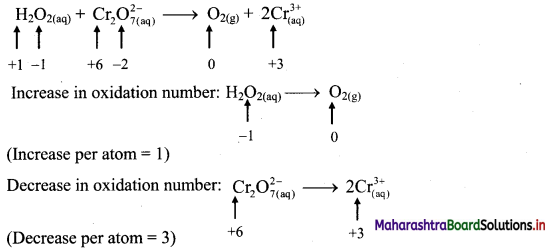Since there are two Cr atoms, the net decrease in oxidation number is 6. In order to make the net increase and decrease equal, we must take 6 atoms of O i.e., 3H2O2.
$$3 \mathrm{H}_{2} \mathrm{O}_{2(\mathrm{aq})}+\mathrm{Cr}_{2} \mathrm{O}_{7(\mathrm{aq})}^{2-} \longrightarrow 3 \mathrm{O}_{2(\mathrm{~g})}+2 \mathrm{Cr}_{(\mathrm{aq})}^{3+}$$

Step 3: Balance ‘O’ atoms by adding 7H2O to the right-hand side.
$$3 \mathrm{H}_{2} \mathrm{O}_{2(\mathrm{aq})}+\mathrm{Cr}_{2} \mathrm{O}_{7(\mathrm{aq})}^{2-} \longrightarrow 3 \mathrm{O}_{2(\mathrm{~g})}+2 \mathrm{Cr}_{(\mathrm{aq})}^{3+}+7 \mathrm{H}_{2} \mathrm{O}_{(l)}$$

Step 4: The medium is acidic. To make the charges and hydrogen atoms on the two sides equal, add 8H+ on the left-hand side.
$$3 \mathrm{H}_{2} \mathrm{O}_{2(\mathrm{aq})}+\mathrm{Cr}_{2} \mathrm{O}_{7(\mathrm{aq})}^{2-}+8 \mathrm{H}_{(\mathrm{aq})}^{+} \longrightarrow 3 \mathrm{O}_{2(\mathrm{~g})}+2 \mathrm{Cr}_{(\mathrm{aq})}^{3+}+7 \mathrm{H}_{2} \mathrm{O}_{(l)}$$

Step 5: Check two sides for balance of atoms and charges.
Hence, balanced equation:
$$3 \mathrm{H}_{2} \mathrm{O}_{2(\mathrm{aq})}+\mathrm{Cr}_{2} \mathrm{O}_{7(\mathrm{aq})}^{2-}+8 \mathrm{H}_{(\mathrm{aq})}^{+} \longrightarrow 3 \mathrm{O}_{2(\mathrm{~g})}+2 \mathrm{Cr}_{(\mathrm{aq})}^{3+}+7 \mathrm{H}_{2} \mathrm{O}_{(l)}$$

ii. $$\mathrm{Ag}_{(\mathrm{s})}+\mathrm{NO}_{3(\mathrm{q})}^{-} \longrightarrow \mathrm{Ag}_{(\mathrm{aq})}^{+}+\mathrm{NO}_{2(\mathrm{~g})}$$

Step 1: Write skeletal equation and balance the elements other than O and H.
$$\mathrm{Ag}_{(\mathrm{s})}+\mathrm{NO}_{3(\mathrm{q})}^{-} \longrightarrow \mathrm{Ag}_{(\mathrm{aq})}^{+}+\mathrm{NO}_{2(\mathrm{~g})}$$

Step 2: Assign oxidation number to Ag and N. Calculate the increase and decrease in the oxidation number and make them equal.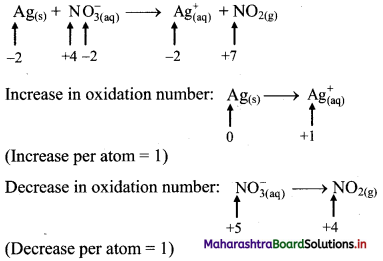Because net increase is equal to net decrease, multiplying coefficients are not required.

Step 3: Balance ‘O’ atoms by adding H2O to the right-hand side.
$$\mathrm{Ag}_{(\mathrm{s})}+\mathrm{NO}_{3(\mathrm{aq})}^{-} \longrightarrow \mathrm{Ag}_{(\mathrm{aq})}^{+}+\mathrm{NO}_{2(\mathrm{~g})}+\mathrm{H}_{2} \mathrm{O}_{(l)}$$

Step 4: The medium is acidic. To make the charges and hydrogen atoms on the two sides equal, add 2H+ on the left-hand side.
$$\mathrm{Ag}_{(\mathrm{s})}+\mathrm{NO}_{3(\mathrm{aq})}^{-}+2 \mathrm{H}_{(\mathrm{aq})}^{+} \longrightarrow \mathrm{Ag}_{(\mathrm{aq})}^{+}+\mathrm{NO}_{2(\mathrm{~g})}+\mathrm{H}_{2} \mathrm{O}_{(l)}$$
Thus, the equation is balanced with respect to the atoms as well as charges.

Step 5: Check two sides for balance of atoms and charges.
Hence, balanced equation: $$\mathrm{Ag}_{(\mathrm{s})}+\mathrm{NO}_{3(\mathrm{aq})}^{-}+2 \mathrm{H}_{(\mathrm{aq})}^{+} \longrightarrow \mathrm{Ag}_{(\mathrm{aq})}^{+}+\mathrm{NO}_{2(\mathrm{~g})}+\mathrm{H}_{2} \mathrm{O}_{(l)}$$

iii. $$\mathrm{Sn}_{(\mathfrak{a q})}^{2+}+\mathrm{IO}_{4(\mathrm{aq})}^{-} \longrightarrow \mathrm{Sn}_{(\mathrm{aq})}^{4+}+\mathrm{I}_{(\mathrm{aq})}^{-}$$

Step 1: Write skeletal equation and balance the elements other than O and H.
$$\mathrm{Sn}_{(\mathfrak{a q})}^{2+}+\mathrm{IO}_{4(\mathrm{aq})}^{-} \longrightarrow \mathrm{Sn}_{(\mathrm{aq})}^{4+}+\mathrm{I}_{(\mathrm{aq})}^{-}$$

Step 2: Assign oxidation number to Sn and I. Calculate the increase and decrease in the oxidation number and make them equal.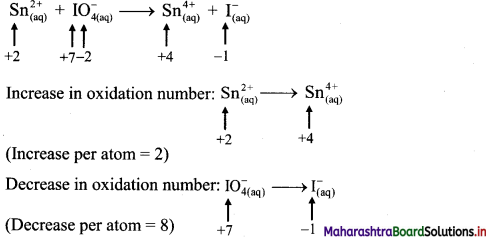To make the net increase and decrease equal, we must take 4 atoms of Sn.
$$4 \mathrm{Sn}_{(\mathrm{aq})}^{2+}+\mathrm{IO}_{4(\mathrm{aq})}^{-} \longrightarrow 4 \mathrm{Sn}_{(\mathrm{aq})}^{4+}+\mathrm{I}_{(\mathrm{aq})}^{-}$$

Step 3: Balance ‘O’ atoms by adding 4H2O to the right-hand side.
$$4 \mathrm{Sn}_{(\mathrm{aq})}^{2+}+\mathrm{IO}_{4(\mathrm{aq})}^{-} \longrightarrow 4 \mathrm{Sn}_{(\text {aq })}^{4+}+\mathrm{I}_{(\text {aq })}^{-}+4 \mathrm{H}_{2} \mathrm{O}_{(l)}$$

Step 4: The medium is acidic. To make the charges and hydrogen atoms on the two sides equal, add 8H+ on the left-hand side.
$$4 \mathrm{Sn}_{(\mathrm{aq})}^{2+}+\mathrm{IO}_{4(\mathrm{aq})}^{-}+8 \mathrm{H}_{(\mathrm{aq})}^{+} \longrightarrow 4 \mathrm{Sn}_{(\mathrm{aq})}^{4+}+\mathrm{I}_{(\mathrm{aq})}^{-}+4 \mathrm{H}_{2} \mathrm{O}_{(l)}$$

Step 5: Check two sides for balance of atoms and charges.
Hence, balanced equation: $$4 \mathrm{Sn}_{(\mathrm{aq})}^{2+}+\mathrm{IO}_{4(\mathrm{aq})}^{-}+8 \mathrm{H}_{(\mathrm{aq})}^{+} \longrightarrow 4 \mathrm{Sn}_{(\mathrm{aq})}^{4+}+\mathrm{I}_{(\mathrm{aq})}^{-}+4 \mathrm{H}_{2} \mathrm{O}_{(l)}$$

iv. $$\mathrm{MnO}_{4(\mathrm{aq})}^{-}+\mathrm{IO}_{3(\mathrm{aq})}^{-} \longrightarrow \mathrm{MnO}_{2(\mathrm{~s})}+\mathrm{IO}_{4(\mathrm{aq})}^{-}$$

Step 1: Write skeletal equation and balance the elements other than O and H.
$$\mathrm{MnO}_{4(\mathrm{aq})}^{-}+\mathrm{IO}_{3(\mathrm{aq})}^{-} \longrightarrow \mathrm{MnO}_{2(\mathrm{~s})}+\mathrm{IO}_{4(\mathrm{aq})}^{-}$$

Step 2: Assign oxidation number to Mn and I. Calculate the increase and decrease in the oxidation number and make them equal.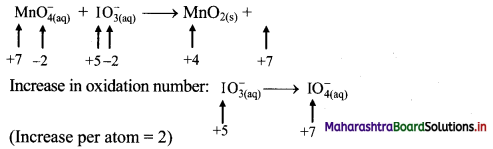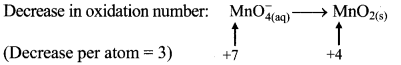To make the net increase and decrease equal, we must take 2 atoms of Mn and 3 atoms of I.
$$2 \mathrm{MnO}_{4(a q)}^{-}+3 \mathrm{IO}_{3(\mathrm{aq})}^{-} \longrightarrow 2 \mathrm{MnO}_{2(\mathrm{~s})}+3 \mathrm{IO}_{4(\mathrm{aq})}^{-}$$

Step 3: Balance ‘O’ atoms by adding 1H2O to the right-hand side.
$$2 \mathrm{MnO}_{4(\mathrm{aq})}^{-}+3 \mathrm{IO}_{3(\mathrm{aq})}^{-} \longrightarrow 2 \mathrm{MnO}_{2(\mathrm{~s})}+3 \mathrm{IO}_{4(\mathrm{aq})}^{-}+\mathrm{H}_{2} \mathrm{O}_{(l)}$$

Step 4: The medium is acidic. To make the charges and hydrogen atoms on the two sides equal, add 2H on the left-hand side.
$$2 \mathrm{MnO}_{4(\mathrm{aq})}^{-}+3 \mathrm{IO}_{3(\mathrm{aq})}^{-}+2 \mathrm{H}_{(\mathrm{aq})}^{+} \longrightarrow 2 \mathrm{MnO}_{2(\mathrm{~s})}+3 \mathrm{IO}_{4(\mathrm{aq})}^{-}+\mathrm{H}_{2} \mathrm{O}_{(l)}$$

Step 5: Check two sides for balanced of atoms and charges.
Hence, balanced equation: $$2 \mathrm{MnO}_{4(\mathrm{aq})}^{-}+3 \mathrm{IO}_{3(\mathrm{aq})}^{-}+2 \mathrm{H}_{(\mathrm{aq})}^{+} \longrightarrow 2 \mathrm{MnO}_{2(\mathrm{~s})}+3 \mathrm{IO}_{4(\mathrm{aq})}^{-}+\mathrm{H}_{2} \mathrm{O}_{(l)}$$

Question 32.
Balance the following redox equation in basic medium by oxidation number method: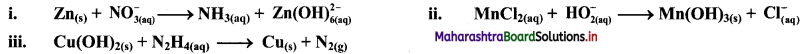i. $$\mathrm{Zn}_{(\mathrm{s})}+\mathrm{NO}_{3(\mathrm{aq})}^{-} \longrightarrow \mathrm{NH}_{3(\mathrm{aq})}+\mathrm{Zn}(\mathrm{OH})_{6(\mathrm{aq})}^{2-}$$

Step 1: Write skeletal equation and balance the elements other than 0 and H.
$$\mathrm{Zn}_{(\mathrm{s})}+\mathrm{NO}_{3(\mathrm{aq})}^{-} \longrightarrow \mathrm{NH}_{3(\mathrm{aq})}+\mathrm{Zn}(\mathrm{OH})_{6(\mathrm{aq})}^{2-}$$

Step 2: Assign oxidation number to Zn and N. Calculate the increase and decrease in the oxidation number and make them equal.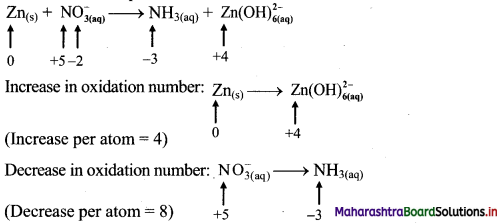To make the net increase and decrease equal, we must take 2 atoms of Zn.
$$2 \mathrm{Zn}_{(\mathrm{s})}+\mathrm{NO}_{3(\mathrm{aq})}^{-} \longrightarrow \mathrm{NH}_{3(\mathrm{aq})}+2 \mathrm{Zn}(\mathrm{OH})_{6(\mathrm{aq})}^{2-}$$

Step 3: Balance ‘O’ atoms by adding 9H2O to the left-hand side.
$$2 \mathrm{Zn}_{(\mathrm{s})}+\mathrm{NO}_{3(\mathrm{aq})}^{-}+9 \mathrm{H}_{2} \mathrm{O}_{(l)} \longrightarrow \mathrm{NH}_{3(\mathrm{aq})}+2 \mathrm{Zn}(\mathrm{OH})_{6(\mathrm{aq})}^{2-}$$

Step 4: The medium is basic. To make hydrogen atoms on the two sides equal, add 3H on the right-hand side.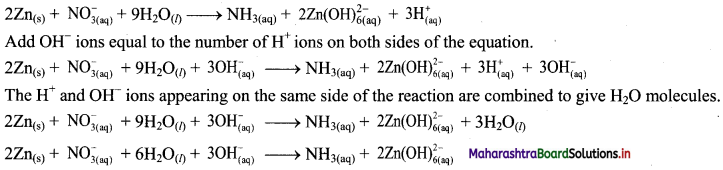Step 5: Check two sides for balance of atoms and charges.
Hence, balanced equation: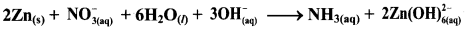ii. $$\mathrm{MnCl}_{2(\mathrm{aq})}+\mathrm{HO}_{2(\mathrm{aq})}^{-} \longrightarrow \mathrm{Mn}(\mathrm{OH})_{3(\mathrm{~s})}+\mathrm{Cl}_{(\mathrm{aq})}^{-}$$

Step 1: Write skeletal equation and balance the elements other than O and H.
$$\mathrm{MnCl}_{2(\mathrm{aq})}+\mathrm{HO}_{2(\mathrm{aq})}^{-} \longrightarrow \mathrm{Mn}(\mathrm{OH})_{3(\mathrm{~s})}+\mathrm{Cl}_{(\mathrm{aq})}^{-}$$

Step 2: Assign oxidation number to Mn and O. Calculate the increase and decrease in the oxidation number and make them equal.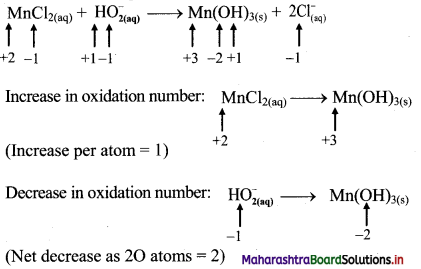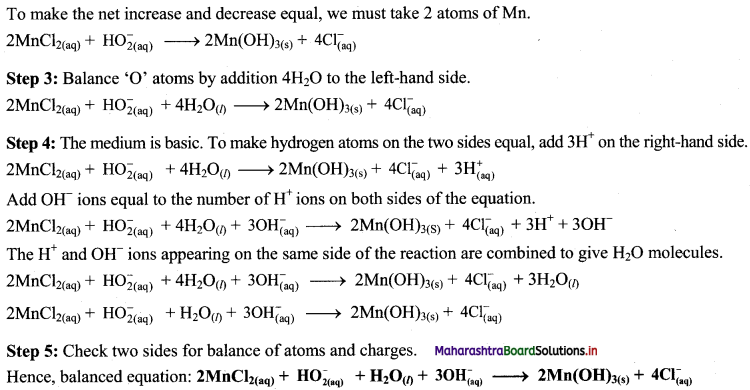iii. Cu(OH)2(s) + N2H4(aq) → Cu(s) + N2(g)
Step 1: Write skeletal equation and balance the elements other that O and H.
Cu(OH)2(s) + N2H4(aq) → Cu(s) + N2(g)
Step 2: Assign oxidation number to Cu and N. Calculate the increase and decrease in the oxidation number and make them equal.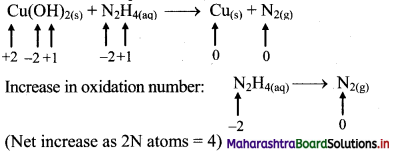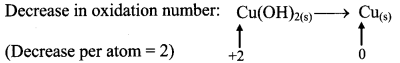To make the net increase and decrease equal, we must take 2 atoms of Cu.
2Cu(OH)2(s) + N2H4(aq) → 2Cu(s) + N2(g)
Step 3: Balance ‘O’ atoms by adding 4H20 to the right-hand side.
2Cu(OH)2(s) + N2H4(aq) → 2Cu(s) + N2(g) + 4H2O(l)
Step 4: The equation is balanced for both atoms as well as charges.
Step 5: Check two sides for balance of atoms and charges.
Hence, balanced equation: 2Cu(OH)2(s) + N2H4(aq) → 2Cu(s) + N2(g) + 4H2O(l)Question 33.
Describe the steps involved in balancing redox reactions by ion electron method (Half reaction method).
In this method two half equations are balanced separately and then added together to give balanced equation. Following steps are involved.

• Step 1: Write unbalanced equation for the redox reaction, assign oxidation number to all the atoms in the reactants and products. Divide the equation into two half equations. One half equation involves increase in oxidation number and another involves decrease in oxidation number (Write two half equations separately).
• Step 2: Balance the atoms except O and H in each half equation. Balance oxygen atom by adding H2O to the side with less O atoms.
• Step 3: Balance H atoms by adding H+ ions to the side having less H atoms.
• Step 4: Balance the charges by adding appropriate number of electrons to the right side of oxidation half equation and to the left of reduction half equation.
• Step 5: Multiply half equation by suitable factors to equalize the number of electrons in two half equations. Add two half equations and cancel the number of electrons on both sides of equation.
• Step 6: If the reaction occurs in basic medium then add OH ions, equal to number of H+ ions on both sides of equation. The H+ and OH ions on same side of equation combine to give H2O molecules.
• Check that the equation is balanced in both, the atoms and the charges.

Question 34.
Balance the following unbalanced equation (in acidic medium) by ion electron method (half reaction method).
$$\mathrm{Mn}_{(\mathrm{aq})}^{2+}+\mathrm{ClO}_{3(\mathrm{qq})}^{-} \longrightarrow \mathrm{MnO}_{2(\mathrm{~s})}+\mathrm{ClO}_{2(\mathrm{aq})}$$
Step 1: Write imbalanced equation for the redox reaction. Assign oxidation number to all the atoms in reactants and products. Divide the equation into two half equations.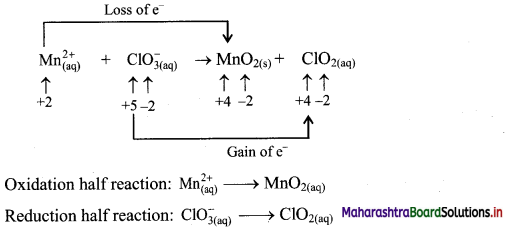Step 2: Balance the atoms except O and H in each half equation. Balance half equations for O atoms by adding H2O to the side with less O atoms. Add 2H2O to left side of oxidation half equation and 1H2O to the right side of reduction half equation.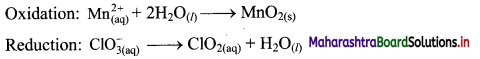Step 3: Balance H atoms by adding H+ ions to the side with less H. Hence add 4H+ ions to the right side of oxidation half equation and 2H+ ions to the left side of reduction half equation.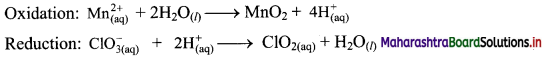Step 4: Now add 2 electrons to the right side of oxidation half equation and 1 electron to the left side of reduction half equation to balance the charges.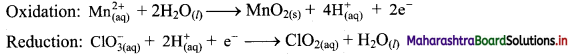Step 5: Multiply reduction half equation by 2 to equalize number of electrons in two half equations. Then add two half equation.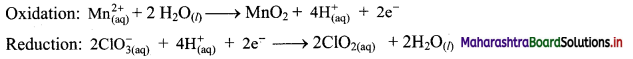$$\mathrm{Mn}_{(\mathrm{aq})}^{2+}+2 \mathrm{ClO}_{3(\mathrm{aq})}^{-} \longrightarrow \mathrm{MnO}_{2(\mathrm{~s})}+2 \mathrm{ClO}_{2(\mathrm{aq})}$$
The equation is balanced in terms of number of atoms and the charges.
Hence, balanced equation: $$\mathrm{Mn}_{(\mathrm{aq})}^{2+}+2 \mathrm{ClO}_{3(\mathrm{aq})}^{-} \longrightarrow \mathrm{MnO}_{2(\mathrm{~s})}+2 \mathrm{ClO}_{2(\mathrm{aq})}$$

Question 35.
Balance the following unbalanced equation by ion electron method (half reaction method).
$$\mathrm{H}_{2} \mathrm{O}_{2(\mathrm{aq})}+\mathrm{ClO}_{4(\mathrm{aq})}^{-} \longrightarrow \mathrm{ClO}_{2(\mathrm{aq})}^{-}+\mathrm{O}_{2(\mathrm{~g})}$$
Step 1: Write unbalanced equation for the redox reaction. Assign oxidation number to all the atoms in reactants and products. Divide the equation into two half equations.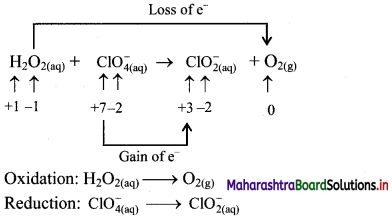Step 2: Balance the atoms except O and H in each half equation. Balance the half equation for O atoms by adding H2O to the side with less O atoms. Hence, add 2H2O to the right side of reduction half equation and none to the oxidation half equation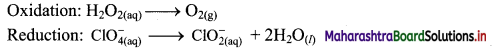Step 3: Balance H atoms by adding H+ ions to the side with less H. Hence add 2H+ ions to the right side of oxidation half equation and 4H+ ions to the left side of reduction half equation.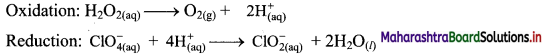Step 4: Add 2 electrons to the right side of oxidation half equation and 4 electrons to the left side of reduction half equation to balance the charges.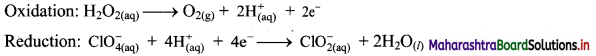Step 5: Multiply oxidation half equation by 2 to equalize the number of electrons and then add two half equations.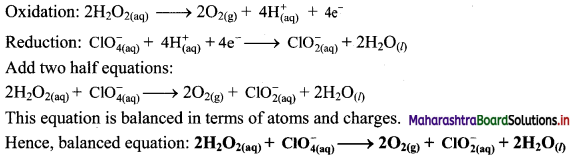Question 36.
Balance the following redox equations by ion-electron (half reaction method).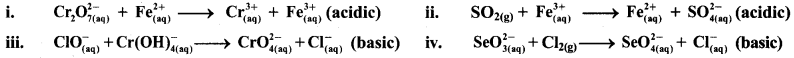i. $$\mathrm{Cr}_{2} \mathrm{O}_{7(\mathrm{aq})}^{2-}+\mathrm{Fe}_{(\mathrm{aq})}^{2+} \longrightarrow \mathrm{Cr}_{(\mathrm{aq})}^{3+}+\mathrm{Fe}_{(\mathrm{aq})}^{3+}(\text { acidic })$$
Step 1: Write unbalanced equation for the redox reaction. Assign oxidation number to all the atoms in reactants and products. Divide the equation into two half equations.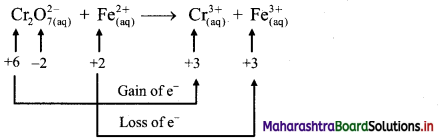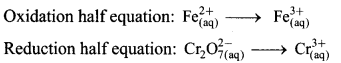Step 2: Balance the atoms except O and H in each half equation. Balance half equation for O atoms by adding 7H2O to the right side of reduction half equation.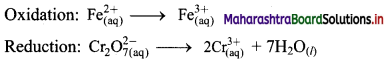Step 3: Balance H atoms by adding H+ ions to the side with less H. Hence, add 14H+ ions to the left side of reduction half equation.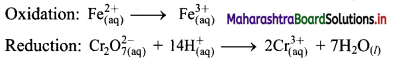Step 4: Now add 1 electron to the right side of oxidation half equation and 6 electrons to the left side of reduction half equation to balance the charges.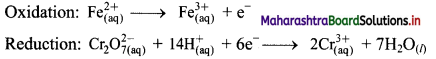Step 5: Multiply oxidation half equation by 6 to equalize number of electrons in two half equations. Then add two half equation.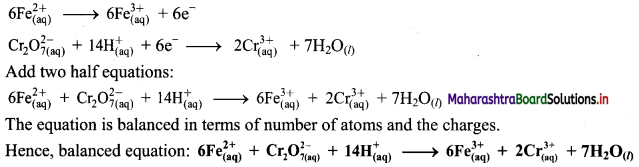ii. $$\mathrm{SO}_{2(\mathrm{~g})}+\mathrm{Fe}_{(\mathrm{aq})}^{3+} \longrightarrow \mathrm{Fe}_{(\mathrm{aq})}^{2+}+\mathrm{SO}_{4(\mathrm{aq})}^{2-}(\text { acidic })$$
Step 1 : Write unbalanced equation for the redox reaction. Assign oxidation number to all the atoms in reactants and products in them. Divide the equation into two half equations.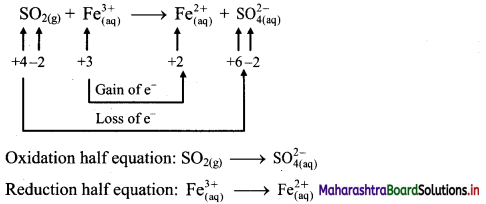Step 2: Balance the atoms except O and H in each half equation. Balance half equation for O atoms by adding 2H2O to the left side of oxidation half equation.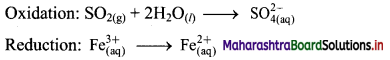Step 3: Balance H atoms by adding H+ ions to the side with less H. Hence, add 4H+ ions to the right side of oxidation half equation.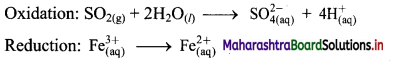Step 4: Now add 2 electrons to the right side of oxidation half equation and 1 electron to the left side of reduction half equation to balance the charges.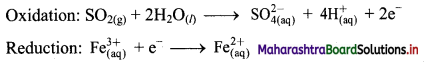Step 5: Multiply reduction half equation by 2 to equalize number of electrons in two half equations. Then add two half equation.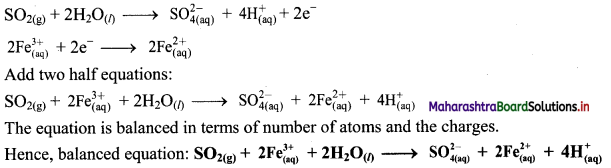iii. $$\mathrm{ClO}_{(\mathrm{aq})}^{-}+\mathrm{Cr}(\mathrm{OH})_{4(\mathrm{aq})}^{-} \longrightarrow \mathrm{CrO}_{4(\mathrm{aq})}^{2-}+\mathrm{Cl}_{(\mathrm{aq})}^{-} \text {(basic) }$$
Step 1: Write unbalanced equation for the redox reaction: Assign oxidation number to all the atoms in reactants and products. Divide the equation into two half equations.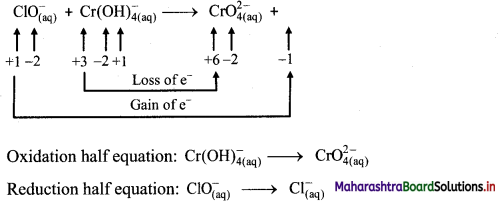Step 2: Balance the atoms except O and H in each half equation. Balance half equation for O atoms by adding 1H2O to the right side of reduction half equation.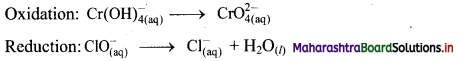Step 3: Balance H atoms by adding H+ ions to the side with less H. Hence, add 4H+ ions to the right side of oxidation half equation and 2H+ ions to the left side of reduction half equation.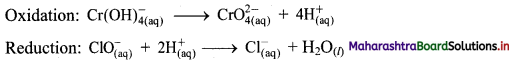Step 4: Now add 3 electrons to the right side of oxidation half equation and 2 electrons to the left side of reduction half equation to balance the charges.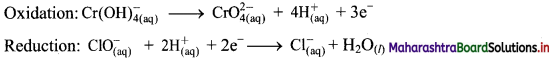Step 5: Multiply oxidation half equation by 2 and reduction half equation by 3 to equalize number of electrons in two half equations. Then add two half equation.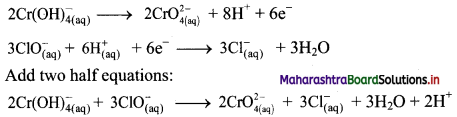Step 6: The reaction takes place in basic medium. 20H ions, equal to the number of H+ ions (2H+ ions) are added on both sides of the equation.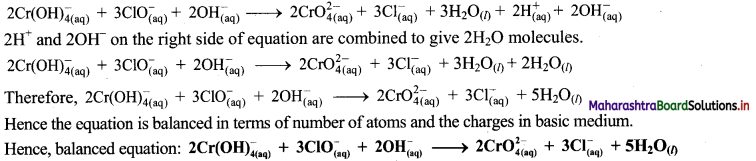iv. $$\mathrm{SeO}_{3(\mathrm{aq})}^{2-}+\mathrm{Cl}_{2(\mathrm{~g})} \longrightarrow \mathrm{SeO}_{4(a q)}^{2-}+\mathrm{Cl}_{(\mathrm{aq})}^{-} \text {(basic) }$$
Step 1: Write unbalanced equation for the redox reaction. Assign oxidation number to all the atoms in reactants and products in them. Divide the equation into two half equations.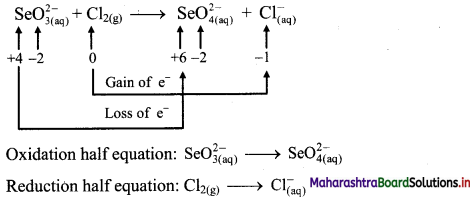Step 2: Balance the atoms except O and H in each half equation. Balance half equation for O atoms by adding H2O to the left side of oxidation half equation.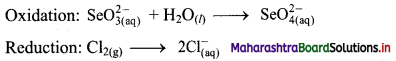Step 3: Balance H atoms by adding H+ ions to the side with less H. Hence, add 2H+ ions to the right side of oxidation half equation.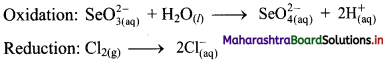Step 4: Now add 2 electrons to the right side of oxidation half equation and 2 electrons to the left side of reduction half equation to balance the charges.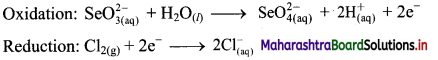Step 5: There is equal number of electrons in two half equations. Add two half equation.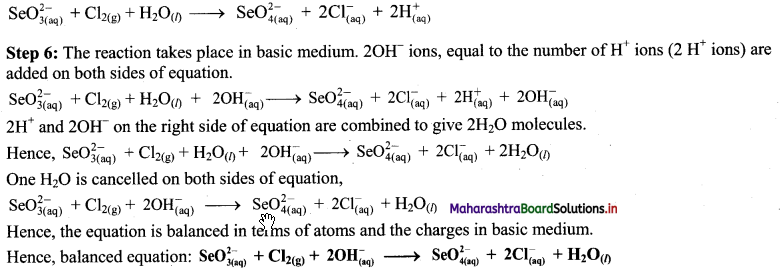Question 37.
Explain displacement reaction in terms of redox reaction by giving example.
i. Displacement reaction can be looked upon as redox reaction. Consider the following displacement reaction.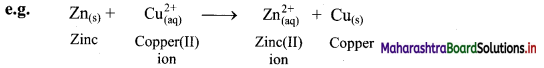ii. Here, Zn gets oxidized to Zn2+ ion and Cu2+ ions get reduced to metallic Cu. A direct transfer of electron from zinc atom to cupric ions takes place in this case.Question 38.
Explain construction of Daniel cell.

• The zinc and copper plates are connected by an electric wire through a switch and voltmeter.
• The solution in two containers are connected by salt bridge (U-shaped glass tube containing a gel of KCl or NH4NO3 in agar-agar).
• When switch is on, electrical circuit is complete as indicated by the deflection in the voltmeter.
• The circuit has two parts, one in the form of electrical wire which allows the flow of electrons and the other in the form of two solutions joined by salt bridge. In solution part of the circuit, the electric current is carried by movement of ions.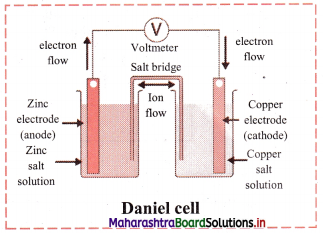Question 39.
Explain working of Daniel cell.
i. When a circuit is complete, the zinc atoms on zinc plates spontaneously lose electrons which are picked up in the external circuit.
ii. The electrons flow from the zinc plate to copper plate through wire.
iii. Cu2+ ions in the second container receive these electrons through the copper plate and are reduced to copper atoms which get deposited on the copper plate.
iv. Here, zinc plate acts as anode (negative electrode) and the copper plate acts as cathode (positive electrode).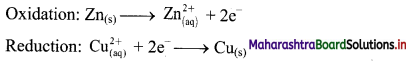v. Thus, when two half reactions, namely, oxidation and reduction, are allowed to take place in separate containers and provision is made for completing the electrical circuit, electron transfer take place through the circuit.
vi. This results in flow of electric current in the circuit as indicated by deflection in voltmeter.
vii. Thus, in Daniel cell, electricity is generated by redox reaction.

Question 40.
Draw a neat and labelled diagram of Daniel cell.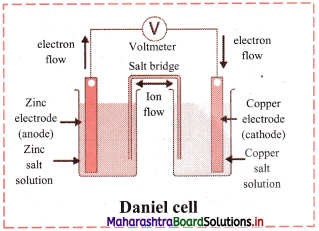Question 41.
Chalcopyrite (CuFeS2) is a common ore of copper. Since it has low concentration of copper, the ore is first concentrated through froth floatation process. The concentrated ore is then heated strongly with silicon dioxide (silica) and oxygen in a furnace. The product obtained, copper(I) sulphide, is further converted to copper (99.5% pure) with a final blast of air (O2) during which sulphur dioxide is released as a by-product.
i. Write a balanced reaction for the extraction of copper from copper(I) sulphide.
ii. Which species undergoes an increase in the oxidation state?
iii. Which species accepts electrons?
i. Cu2S + O2 → 2Cu + SO2
ii. Sulphur undergoes an increase in the oxidation state from -2 (in Cu2S) to +4 (in SO2).
iii. Copper accepts one electron and undergoes a decrease in the oxidation state from +1(in Cu2S) to 0 (in Cu). Oxygen accepts two electrons and undergoes a decrease in the oxidation state from 0 (in O2) to -2 (in SO2).Question 42.
Consider the elements: Cs, Ne, I and F
i. Identify the element that exhibits only negative oxidation state.
ii. Identify the element that exhibits only positive oxidation state.
iii. Identify the element that exhibits both negative as well as positive oxidation state.
iv. Identify the element that exhibits neither the negative nor the positive oxidation state.
i. F: It is most electronegative. It shows only a negative oxidation state of -1.
ii. Cs: Alkali metals have only one electron in their valence shell and hence, exhibits only positive (+1) oxidation state.
iii. I: Because of the presence of 7 electrons in its valence shell, I shows negative oxidation state of -1 (to have stable noble gas configuration) and positive oxidation numbers of +1, +3, +5 and +7 because of the presence of d-orbitals.
iv. Ne: It is an inert gas and therefore, does not exhibit negative or positive oxidation state.

Multiple Choice Questions

1. Loss of electrons means ………….
(A) reduction
(B) oxidation
(C) precipitation
(D) complexometry
(B) oxidation

2. Reduction involves ……………
(A) gain of electrons
(C) increase in oxidation number
(D) loss of electron
(A) gain of electrons

3. Which of the following statement is INCORRECT?
(A) Oxidant is a substance which increases the oxidation number of other substance.
(B) Reductant is a substance which decreases the oxidation number of other substance.
(C) The oxidation number of oxidant decreases.
(D) In oxidation, there is decrease in oxidation number.
(D) In oxidation, there is decrease in oxidation number.

4. Which of the following is an example of oxidation process?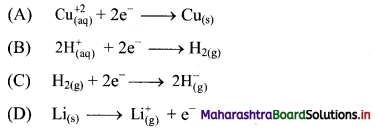(D) $$\mathrm{Li}_{(\mathrm{s})} \longrightarrow \mathrm{Li}_{(\mathrm{g})}^{+}+\mathrm{e}^{-}$$5. Which is the best description of the behaviour of chlorine in the reaction?
H2O + Cl2 → HOCl + HCl
(A) Neither oxidized not reduced
(B) Both oxidised and reduced
(C) Oxidised only
(D) Reduced only
(B) Both oxidised and reduced

6. In the reaction,
$$\begin{array}{r} 3 \mathrm{Br}_{2}+6 \mathrm{CO}_{3}^{2-}+3 \mathrm{H}_{2} \mathrm{O} \longrightarrow \ 5 \mathrm{Br}^{-}+\mathrm{BrO}_{3}^{-}+6 \mathrm{HCO}_{3}^{-} \end{array}$$ …………..
(A) Br2 is oxidised and carbonate is reduced
(B) bromine is reduced and water is oxidised
(C) bromine is neither reduced nor oxidised
(D) bromine is both reduced and oxidised
(D) bromine is both reduced and oxidised

7. A chemical reaction in which oxidation and reduction processes takes place simultaneously is known as ………… reaction.
(A) redox
(B) precipitation
(C) complexometric
(D) titration
(A) redox

8. Which of the following is a redox reaction?
(A) NaCl + KNO3 → NaNO3 + KCl
(B) CaC2O4 + 2HCl → CaCl2 + H2C2O4
(C) Mg(OH)2 + 2NH4Cl → MgCl2 + 2NH4OH
(D) Zn + 2AgCN → 2Ag + Zn(CN)2
(D) Zn + 2AgCN → 2Ag + Zn(CN)2

9. Oxidation number of metal ion is always …………..
(A) positive
(B) negative
(C) zero
(D) non zero
(A) positive10. The oxidation number of oxygen in peroxide is …………..
(A) -2
(B) -1
(C) +1
(D) +2
(B) -1

11. The oxidation number of oxygen is …………. in oxygen difluoride.
(A) -2
(B) -1
(C) +2
(D) +1
(C) +2

12. Oxidation number of carbon in CH2F2 is ………….
(A) +1
(B) -1
(C) 0
(D) +2
(C) 0

13. In calcium hydride (CaH2), the oxidation number of hydrogen is ………….
(A) +1
(B) -1
(C) +2
(D) -2
(B) -1

14. The element with atomic number 9 can exhibit oxidation state of …………..
(A) +1
(B) +3
(C) -1
(D) +5
(C) -115. The highest and lowest oxidation states possible for Te (group 16) are ……………
(A) +6, -2
(B) +6, 0
(C) +4, -4
(D) +6, -6
(A) +6, -2

16. What is the oxidation state of S in Na2S2 ?
(A) +1
(B) -2
(C) -1
(D) 0
(C) -1

17. The oxidation state of S in S2O82- is ………….
(A) +2
(B) + 4
(C) +6
(D) + 7
(D) + 7

18. The oxidation state of phosphorus in Ba(H2PO2)2 is …………..
(A) +3
(B) +2
(C) +1
(D) -1
(C) +1

19. Amongst the following, identify the species having an atom with +6 oxidation state.
(A) $$\mathrm{MnO}_{4}^{-}$$
(B) $$\mathrm{Cr}(\mathrm{OH})_{3}^{6-}$$
(C) $$\mathrm{NiF}_{6}^{2-}$$
(D) CrO2Cl2
(D) CrO2Cl220. In which of the following compounds, the oxidation number of carbon is NOT zero?
(A) (CHCl)2
(B) HCHO
(C) CH3COOH
(D) CH2Cl2
(C) CH3COOH

21. The oxidation number of S in S8, S2F2 and H2S respectively are ………….
(A) 0, +1, -2
(B) +2, +1, -2
(C) 0, +1, +2
(D) +2, +1, -2
(A) 0, +1, -2

22. The coefficients x, y, and z in the following balanced equation
xZn + $$\mathrm{yNO}_{3}^{-}$$ + 10H+ → zZn2+ + $$\mathrm{NH}_{4}^{+}$$ + 3H2O are …………..
(A) 4, 1, 4
(B) 2, 2, 2
(C) 4, 2, 4
(D) 4, 4, 4
(A) 4, 1, 4

23. For the redox reaction:
$$\mathrm{MnO}_{4}{ }^{-}+\mathrm{C}_{2} \mathrm{O}_{4}^{2-}+\mathrm{H}^{+} \longrightarrow \mathrm{Mn}^{2+}+\mathrm{CO}_{2}+\mathrm{H}_{2} \mathrm{O}$$
The CORRECT coefficients of the reactants in the balanced reaction are ……………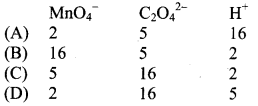(A)

24. In the reaction
3CuO + 2NH3 → N2 + 3H2O + 3Cu
the change of NH3 to N2 involve ……………..
(A) loss of 6 electrons per mol of N2
(B) loss of 3 electrons per mol of N2
(C) gain of 6 electrons per mole N2
(D) gain of 3 electrons per mole N2
(A) loss of 6 electrons per mol of N225. When KMnO4 acts as an oxidizing agent and ultimately forms $$\mathrm{MnO}_{4}^{2-}$$, MnO2, Mn2O3, and Mn2+, then the number of electrons transferred in each case is …………..
(A) 4, 3, 1, 5
(B) 1, 5, 3, 7
(C) 1, 3, 4, 5
(D) 3, 5, 7, 1